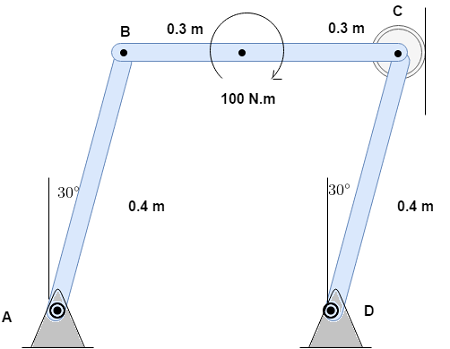# Determine the force which member CD exerts on the pin at C.

## Question:

Determine the force which member CD exerts on the pin at C.## Moment

The moment is the agent which tries to produce an angular rotation inside a body about a fixed point. Its magnitude can be calculated by the multiplying magnitude of the force and its perpendicular distance from the point about which moment has to figure out.

Become a Study.com member to unlock this answer! Create your account

Given data:

• The torque applied is: {eq}T = 100\;{\rm{N}} \cdot {\rm{m}} {/eq}
• The angle is: {eq}\alpha = 30^\circ {/eq}

Assume the force in...Torque in Physics: Equation, Examples & Problems

from

Chapter 3 / Lesson 13
32K

After watching this video, you will be able to explain what torque is and use an equation to calculate torque in simple situations. A short quiz will follow.